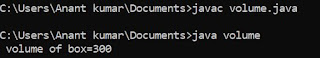## Thursday, 21 June 2018

### How to write program to find average of an array of 5 elements

/* Avg of an array */
# include <stdio.h>
void main ()
{
int a,i,avg ;
int sum = 0 ;
clrscr () ;
for ( i=o ; i<5 ; i++ )
{
printf ("\n Enter a number:") ;
scanf ("%d"< & a[i]) ;
}
for ( i=0 ; i<5 ; i++ )
sum = sum + a[i] ;
avg = sum / 5 ;
printf ("\n avg= %d", avg) ;
getch () ;
}

DRY RUN ;

sum =0 i=0 0<5
a = 11
i=0 0<5 (TRUE)   i++ = 1
sum = sum + a[i]

similarly
for  i = 1,2,3,4  having true

for i = 5 ( 5<5 ) false

#### 1 comment:

1.It is actually a great and helpful piece of information. I am satisfied that you simply shared this helpful information with us. Please stay us informed like this. Thanks for sharing. Guest Posting Sites List 2018

## Featured post

### Program to find Volume of given box in java

class volume { public static void main (String args []) { int l=5,b=6,h=10; int volume; volume=l*b*h; System.out.println (" vol...